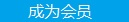您可以捐助，支持我们的公益事业。 1元 10元 50元 认证码：必填求知 文章 文库 Lib 视频 Code iProcess 课程 角色 咨询 工具 火云堂 讲座吧 建模者要资料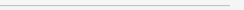订阅捐助
TensorFlow.js、迁移学习与AI产品创新之道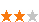1157 次浏览     评价： 好 中 差
2018-5-11

 编辑推荐: 本文来自于网络，全文从技术聊到产品的玩法，设计师／产品经理只有懂得技术的新特性，才能为产品融入新的玩法。

1 概念篇

1.1 tensors

TensorFlow.js 把 N 维数组都统称为 tensor ，为方便理解，见下图。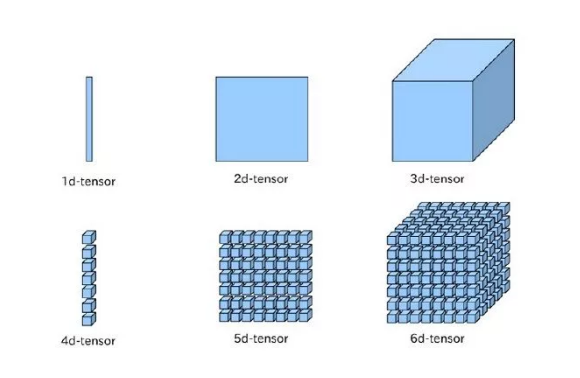var shape=[2,3]; var a=tf.tensor([1,2,3,4,4,5],shape); a.print()

 var a=tf.tensor([[1,2,3],[4,4,5]]); a.print()

 var a=tf.scalar(4); a.print(); var b=tf.tensor1d([0,2,3,4]); b.print(); var c=tf.tensor2d([[0,3],[4,5]]); c.print();

TensorFlow.js还提供了直接创建所有值为0或者1的张量（ tensor ），实验下：

 tf.zeros().print(); tf.zeros([2,8]).print(); tf.zeros([2,2,3]).print(); tf.ones().print(); tf.ones([2,8]).print(); tf.ones([2,2,3]).print();

1.2 Variables

Tensors 是不可变的，一旦创建，不能改变其值；而 variables 则可以动态改变其值，主要用于在模型训练期间存储和更新值。

 var initalValues=tf.ones(); initalValues.print(); //biases变量，通过assign方法更新其值 var biases=tf.variable(initalValues); biases.print(); var updatedValues=tf.tensor1d([0,1,2,3,4,5,6,7]); updatedValues.print(); biases.assign(updatedValues); biases.print();

1.3 Operations ( Ops )

 //square var d=tf.tensor2d([[1,2,3],[4,5,6]]); d.print(); var d_squared=d.square(); d_squared.print(); //add var a=tf.tensor2d([[1,2,3],[4,5,6]]); var b=tf.tensor2d([[3,1,9],[14,25,16]]); a.print(); b.print(); var c=a.add(b); c.print(); d.add(b).square().print();

1.4 Models and Layers

Models 相当于 JS 函数的概念，给定一些输入，使用 Ops 来表示模型所做的工作，产生一些期望的输出。 TensorFLow.js 有 2 种创建模型的方法。

 // 定义一个 predict 函数 function predict(input) { // 实现一个数学函数的计算 y = a * x ^ 2 + b * x + c return tf.tidy(() => { const x = tf.scalar(input); const ax2 = a.mul(x.square()); const bx = b.mul(x); const y = ax2.add(bx).add(c); return y; }); } // 定义常量 var a = tf.scalar(2), b = tf.scalar(4), c = tf.scalar(8); // 测试下 predict 函数 predict(1999993).print();

 // y = 3 ^ 2 + 1 var y = tf.tidy(() => { // a, b, 以及 one 将会被清空当 tidy 结束时。 const one = tf.scalar(1); const a = tf.scalar(3); const b = a.square(); console.log('tensors 的数量 (in tidy): ' + tf.memory().numTensors); return b.add(one); }); console.log('tensors 的数量 (outside tidy): ' + tf.memory().numTensors); y.print();

 const x=tf.tensor2d([[0,2,3],[1,2,3]]); const x_squared=x.square(); x.print(); x_squared.print(); console.log(tf.memory().numTensors); x.dispose(); console.log(tf.memory().numTensors); x_squared.dispose(); console.log(tf.memory().numTensors);

tensorFlow.js 还内置了一些 model 的抽象，可以使用 tf.model 来构造一个不含 layer 的模型。 tf 包含的 layer 有 tf.layers.simpleRNN ， tf.layers.gru 和 tf.layers.lstm 等。这里得通过几个小型项目来实践了。

2 官方示例

 http://www.parceljs.io

3 webcam-transfer-learning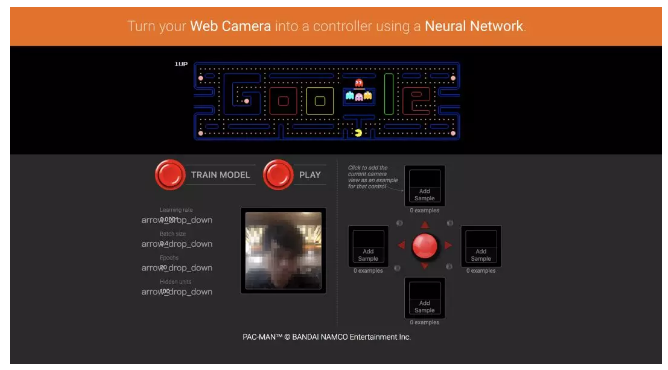3.1 MobileNet

 MobileNets: Efficient Convolutional Neural Networks for Mobile Vision Applications

 https://github.com/fchollet/deep-learning-models/releases/download/v0.6/mobilenet_2_5_224_tf.h5

 pip install tensorflowjs

 tensorflowjs_converter --input_format keras mobilenet_2_5_224_tf.h5 model

 npm install http-server -g

 http-server -p 3000 --cors

 const model = await tf.loadModel(‘https://localhost:3000/model.json');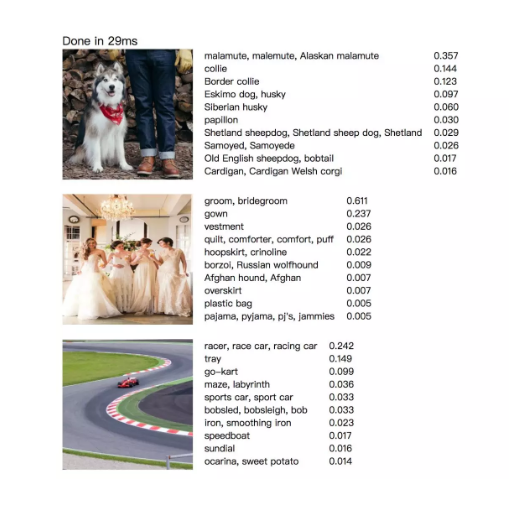3.2 Transfer Learning

webcam-transfer-learning 是一个图像分类问题，将摄像头拍摄的照片与上下左右的动作做关联。主要是训练数据收集：摄像头拍摄，每张图片归一化处理成 shape 为 [1,244,244,3] 的张量，作为训练数据；为此 tensorFlow.js 特地封装了调用 webcam 的相关方法，以方便直接对接到 tensorFLow.js 中使用。并使用 Transfer Learning 迁移学习来减少训练数据的量，达到分类的目的。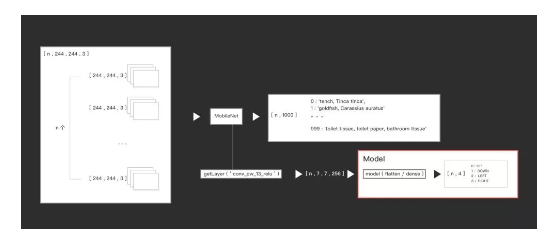3.2.1 预处理

 async function loadMobilenet() { const mobilenet = await tf.loadModel( 'https://storage.googleapis.com/tfjs-models/tfjs/mobilenet_v1_0.25_224/model.json'); console.log(mobilenet.layers) const layer = mobilenet.getLayer(‘conv_pw_13_relu'); console.log(layer.output.shape) return tf.model({ inputs: mobilenet.inputs, outputs: layer.output }); }

 console.log(layer.output.shape)

3.2.2 迁移学习

 model = tf.sequential({ layers: [ tf.layers.flatten({ inputShape: [7, 7, 256] }), tf.layers.dense({ units: ui.getDenseUnits(), activation: 'relu', kernelInitializer: 'varianceScaling', useBias: true }), tf.layers.dense({ units: NUM_CLASSES, kernelInitializer: 'varianceScaling', useBias: false, activation: 'softmax' }) ] });

 model=tf.sequential(); model.add(tf.layers.flatten({inputShape:[12,4]})); nn=model.predict(tf.ones([99,12,4])); console.log(nn.shape); nn.print()

4 基于用户个性化数据的产品

webcam-transfer-learning 游戏给我们提供了一个基于用户个性化数据的玩法。用户可以非常低成本的训练属于自己的图像分类模型，用于各种分类问题。我们可以拓展下，比如识别用户的手势动作，来控制游戏中的人物；识别用户的表情，控制3d人物的表情；识别图像中的人脸数量，自动隐藏所浏览的内容，防止被窥视……甚至 autodraw 、ui2code 、手写字识别等这些应用都可以尝试融入用户个性化的数据再训练的玩法，给予用户掌控权。

―> 采集数据

―> 开始训练

―> 使用用户数据

―> 核心功能

―> 完成任务／得到某个结果。1157 次浏览  评价: 好 中 差订阅捐助
 相关文章 我们该如何设计数据库 数据库设计经验谈 数据库设计过程 数据库编程总结
 相关文档 数据库性能调优技巧 数据库性能调整 数据库性能优化讲座 数据库系统性能调优系列
 相关课程 高性能数据库设计与优化 高级数据库架构师 数据仓库和数据挖掘技术 Hadoop原理、部署与性能调优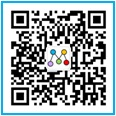每天2个文档/视频 扫描微信二维码订阅
 订阅技术月刊 获得每月300个技术资源